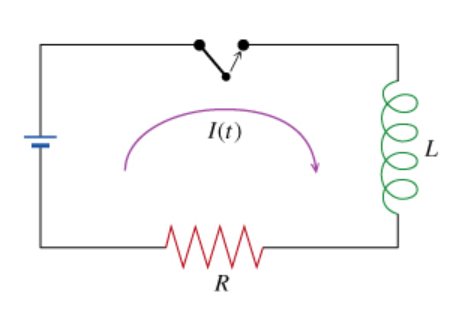# Problem: Consider an L-R circuit as shown in the figure. The battery provides 12.0 V of voltage has resistance R=150Ω. The switch is initially open as shown. At time t=0, the switch is closed. At time t after t=0 the current I(t) flows through the circuit as indicated in the figure. What is the current I(3τ) at a time after t=0 equal to three times the time constant?

###### FREE Expert Solution

Current through the circuit is given by

$\overline{){\mathbf{I}}{\mathbf{\left(}}{\mathbf{t}}{\mathbf{\right)}}{\mathbf{=}}\frac{\mathbf{V}}{\mathbf{R}}{\mathbf{\left(}}{\mathbf{1}}{\mathbf{-}}{{\mathbf{e}}}^{\mathbf{-}\mathbf{R}\mathbf{t}\mathbf{/}\mathbf{L}}{\mathbf{\right)}}}$

Time constant:

$\overline{){\mathbf{\tau }}{\mathbf{=}}{\mathbf{L}}{\mathbf{/}}{\mathbf{R}}}$

V = 12 V###### Problem Details

Consider an L-R circuit as shown in the figure.

The battery provides 12.0 V of voltage has resistance R=150Ω. The switch is initially open as shown. At time t=0, the switch is closed. At time t after t=0 the current I(t) flows through the circuit as indicated in the figure.What is the current I(3τ) at a time after t=0 equal to three times the time constant?